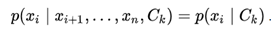# Global AI and Data Science

View Only

## ML Algorithm Naive Bayes

####By Moloy De posted Thu July 09, 2020 11:03 PM

CONDITIONAL PROBABILITY: For two events A and B with P(B) ≠ 0, the Conditional Probability of A given B is defined as P(A|B) = P(A∩B) / P(B).

BAYES’ THEOREM: From the above definition P(A∩B) = P(A|B) P(B). Then for n exhaustive and mutually exclusive sets A1, A2, …, An,

P(Ai|A) = P(A∩Ai) / P(A) = P(Ai|A) P(A) / ∑ P(A∩Ak) = P(Ai|A) P(A) / ∑ P(Ak|A) P(A)

which is Bayes’ Theorem.

NAÏVE BAYES: Consider t classes C1, C2, …, Ct and n observations x1, x2, …, xn. We need to find the class Ck for which P(Ck|x1, x2, …, xn) is maximum over k. Using the definition of Conditional Probability P(Ck|x1, x2, …, xn) = P(Ck, x1, x2, …, xn)/P(x1,x2,…,xn). Now,Now the "naïve" conditional independence assumptions come into play: assume that all features in x are mutually independent, conditional on the category Ck. Under this assumption,under the above independence assumptions, the conditional distribution over the class variable C is:Where Z = P(x) is merely a scaling factor and doesn’t vary over the categories or classes. So the Naïve Bayes algorithm calculates P(Ck|x1, x2, …, xn) for all the classes Ck and chooses the class that has maximum probability.

A BIT OF HISTORY: Naïve Bayes has been studied extensively since the 1960s. It was introduced into the text retrieval community in the early 1960s, and remains a popular baseline method for text categorization, the problem of judging documents as belonging to one category or the other, document categorization such as spam or legitimate, sports or politics, etc., with word frequencies as the features. With appropriate pre-processing, it is competitive in this domain with more advanced methods including support vector machines. It also finds application in automatic medical diagnosis.

In the statistics and computer science literature, naive Bayes models are known under a variety of names, including simple Bayes and independence Bayes. All these names reference the use of Bayes' theorem in the classifier's decision rule, but naïve Bayes is not necessarily a Bayesian method.

SPAM FILTERING: Naive Bayes classifiers work by correlating the use of tokens, typically words, or sometimes other things, with spam and non-spam (ham) e-mails and then using Bayes' theorem to calculate a probability that an email is or is not spam.

Naive Bayes spam filtering is a baseline technique for dealing with spam that can tailor itself to the email needs of individual users and give low false positive spam detection rates that are generally acceptable to users. It is one of the oldest ways of doing spam filtering, with roots in the 1990s.

Variants of the basic technique have been implemented in a number of research works and commercial software products. Many modern mail clients implement Bayesian spam filtering. Users can also install separate email filtering programs. Server-side email filters, such as DSPAM, SpamAssassin, SpamBayes, Bogofilter and ASSP, make use of Bayesian spam filtering techniques, and the functionality is sometimes embedded within mail server software itself. CRM114, oft cited as a Bayesian filter, is not intended to use a Bayes filter in production, but includes the ″unigram″ feature for reference.

NAÏVE BAYES IN PYSPARK: NaiveBayes implements multinomial naive Bayes. It takes an RDD (resilient distributed dataset) of LabeledPoint and an optionally smoothing parameter lambda as input, and output a NaiveBayesModel, which can be used for evaluation and prediction. Note that the Python API does not yet support model save/load but will in the future.

QUESTION I : How justified is the assumption of Conditional Independence in Naïve Bayes?

QUESTION II : Do we need a recurrent procedure in calculating Naïve Bayes probabilities?
#GlobalAIandDataScience
#GlobalDataScience
27 views

Mon September 14, 2020 12:23 AM

Thanks Nitin I think I agree with you. As I practice I try to avoid feature selection as much as possible as it tends to incorporate modeler's subjective judgement. I think a better practice is to let the model do the selection. Say in Linear Regression we may allow maximum possible features and the features that contribute less will have a coefficient close to zero. However, I agree, issues like multicolinearity will stop me from doing so.

Fri September 11, 2020 02:51 PM

In Naive Bayes, we assume that all features have equal weightage. Keeping this in mind, we need not go for feature selection in Naive Bayes classifier.

Tue July 14, 2020 03:45 AM

That's why I was suggesting to combine n-gram tokens with NB.

Tue July 14, 2020 01:24 AM

Thanks. This is what I got from Stanford University site here.

"Naive Bayes is so called because the independence assumptions we have just made are indeed very naive for a model of natural language. The conditional independence assumption states that features are independent of each other given the class. This is hardly ever true for terms in documents. In many cases, the opposite is true. The pairs hong and kong or london and english are examples of highly dependent terms. In addition, the multinomial model makes an assumption of positional independence. The Bernoulli model ignores positions in documents altogether because it only cares about absence or presence. This bag-of-words model discards all information that is communicated by the order of words in natural language sentences. How can NB be a good text classifier when its model of natural language is so oversimplified?"

Tue July 14, 2020 12:28 AM

If the mail length is high, possibly conditional independence can be assumed as an acceptable approximation. Otherwise, n-grams / shingles techniques can also be combined with Naive Bayes to bring in word proximity dependence, if any.